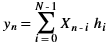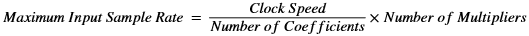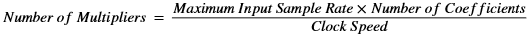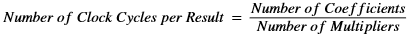# Semi-Parallel FIR Filter

## Versal ACAP DSP Engine Architecture Manual (AM004)

Document ID
AM004
Release Date
2022-09-11
Revision
1.2.1 English

A common filter implementation to exploit available clock cycles, while still achieving moderate to high sample rates, is the semi-parallel FIR filter (also known as folded-hardware). The DSP58 allows creation of optimum filter structures of semi-parallel nature that in turn save resources and potential clock cycles.

In terms of the range of sample rate covered and number of coefficients, the semi-parallel FIR structure fills in the region between parallel FIR filters and sequential FIR filters. The structure implements the general FIR filter equation of a summation of products similar to the one described in the single multiplier MACC FIR filter.Along with achievable clock speed and the number of coefficients (N), the number of multipliers (M) is also a factor in calculating semi-parallel FIR filter performance. The following equation demonstrates that the more multipliers used, the greater the achievable performance of the filter.The maximum input sample rate equation can be rearranged as follows to determine the number of multipliers to use for a particular semi-parallel architecture.The number of clock cycles between each result of the FIR filter is determined by the following equation.